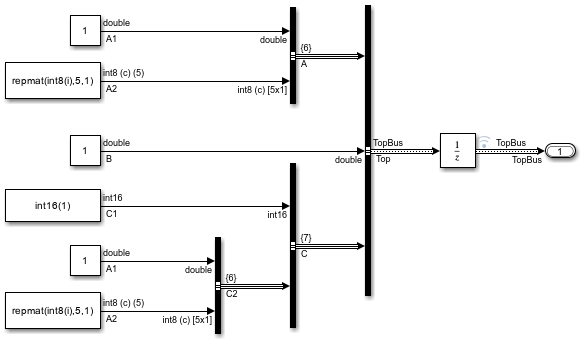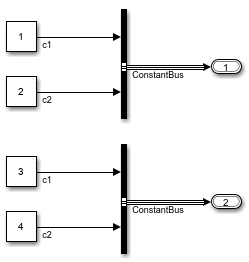Create MATLAB structures that use the same hierarchy and attributes as buses

Description

example

structs = Simulink.Bus.createMATLABStruct(buses) creates one or more MATLAB® structures that have the same hierarchy and attributes as the specified buses. The resulting structures use the ground values of the buses. Use this syntax to create initialization structures for multiple bus ports.

example

structs = Simulink.Bus.createMATLABStruct(buses,values) creates one or more structures that use the specified values.

example

structs = Simulink.Bus.createMATLABStruct(buses,values,dims) creates one or more structures that have the specified dimensions. To create a structure for an array of buses, include the dims argument.

structs = Simulink.Bus.createMATLABStruct(buses,values,dims,scope) creates one or more structures in the data dictionary specified by scope.

Examples

collapse all

Open and simulate model ex_bus_initial_conditions.

open_system('ex_bus_initial_conditions')
sim('ex_bus_initial_conditions');Create a MATLAB structure using bus object Top, which model ex_bus_initial_conditions loads.

Set a value for the field of the mStruct structure that corresponds to bus element A1 of bus A.

mStruct.A.A1 = 3;
mStruct.A
ans =

struct with fields:

A1: 3
A2: [5x1 int8]

Simulink sets the other fields in the structure to the ground values of the corresponding bus elements.

You can use mStruct as the initial condition structure for the Unit Delay block.

Create a MATLAB structure for a bus whose signal elements use a data type other than double. Use a partial structure to specify initialization values for a subset of the elements. When you create the partial structure, match the data types of the fields with the data types of the corresponding elements.

Open and simulate model ex_bus_initial_conditions.

open_system('ex_bus_initial_conditions')
sim('ex_bus_initial_conditions');The C1 signal element that the block labeled Constant5 produces uses the data type int16.

Find the port handle for the Bus Creator block port that produces the Top bus signal.

ph = get_param('ex_bus_initial_conditions/TopBus','PortHandles');

Create a partial structure that specifies values for a subset of the elements in the bus signal created by the TopBus block. To set the value of the C.C1 field, use a typed expression. Match the data type in the expression with the data type of the signal element in the model (int16).

PartialstructForK = struct('B',3,'C',struct('C1',int16(5)));

Create a full structure by using the port handle (ph) for the TopBus block. Override the ground values for the C.C1 and B elements.

outPort = ph.Outport;

The field C.C1 in the output structure continues to use the data type int16.

Open and simulate model ex_bus_initial_conditions.

open_system('ex_bus_initial_conditions')
sim('ex_bus_initial_conditions');Create a partial structure for a subset of bus elements in the bus created by the TopBus block.

PartialStructForK = struct('A',struct('A1',4),'B',3)
PartialStructForK =

struct with fields:

A: [1x1 struct]
B: 3

Create a MATLAB structure using bus object Top, a partial structure, and dimensions for the resulting structure.

('Top',PartialStructForK,[2 3])
structFromBus =

2x3 struct array with fields:

A
B
C

To create initialization structures for multiple bus ports, specify port handles as arguments for Simulink.Bus.createMATLABStruct. The resulting cell array of structures uses ground values.

Open and simulate model ex_two_outports_create_struct.

open_system('ex_two_outports_create_struct')
sim('ex_two_outports_create_struct');Find the port handles for the Bus Creator blocks Bus1 and Bus2.

ph_1 = get_param...
('ex_two_outports_create_struct/Bus Creator','PortHandles');
ph_2 = get_param...
('ex_two_outports_create_struct/Bus Creator1','PortHandles');

Create a MATLAB® structure using an array of port handles.

mStruct =

2x1 cell array

{1x1 struct}
{1x1 struct}

Create a MATLAB structure based on a port that connects to a bus signal. Use a partial structure to specify values for a subset of the bus elements in the bus that connects to the port.

Open and simulate model ex_bus_initial_conditions.

open_system('ex_bus_initial_conditions')
sim('ex_bus_initial_conditions');Find the port handle for the Bus Creator block port that produces the Top bus signal. The Outport handle is the handle that you need.

ph = get_param('ex_bus_initial_conditions/TopBus','PortHandles')
ph =

struct with fields:

Inport: [31.0012 29.0012 41.0012]
Outport: 42.0012
Enable: []
Trigger: []
State: []
LConn: []
RConn: []
Ifaction: []
Reset: []

Create a partial structure for the bus signal created by the TopBus block. You can use a partial structure to specify values for a subset of bus elements.

PartialstructForK = struct('A',struct('A1',4),'B',3)
PartialstructForK =

struct with fields:

A: [1x1 struct]
B: 3

Bus elements represented by structure fields Top.B and Top.A are at the same level in the bus hierarchy. You can use this partial structure to override the ground values for the B and A bus signal elements.

When you create a structure from a bus object or from a bus port, you can use a partial structure as an optional argument.

Create a MATLAB structure by using the port handle (ph) for the TopBus block. Override the ground values for the A.A1 and B bus elements.

outPort = ph.Outport;
mStruct =

struct with fields:

A: [1x1 struct]
B: 3
C: [1x1 struct]

Input Arguments

collapse all

Source of the bus information, specified as a Bus object name, port handle, cell array of Bus object names, or array of port handles.

• If you use a Bus object name, then the Bus object must be in the MATLAB base workspace or the data dictionary used by the model. The data type for a Bus object name is char or string.

• If you use a port handle, then the model must compile successfully before you use this function. The data type for a port handle is double.

• For an array of buses, you cannot use a port handle.

• If you use the dims argument, then for the buses argument, use a Bus object or cell array of Bus objects.

Specifying a cell array of Bus object names or an array of port handles creates multiple structures with one Simulink.Bus.createMATLABStruct call and provides better performance than using separate Simulink.Bus.createMATLABStruct calls to create the structures.

Data Types: double | char | string | struct | cell

Values for a subset of elements in the resulting structure, specified as an empty matrix ([]), partial structure, or cell array. The cell array must contain a partial structure or empty matrix for each specified source of bus information.

For information on creating partial structures, see Create Partial Structures for Initialization.

To use ground values, use an empty matrix.

Data Types: struct | cell

Dimensions of the resulting structure, specified as a vector.

Each dimension element must be an integer that is greater than or equal to 1. If you specify a partial structure for the values argument, each dimension element must be greater than or equal to its corresponding dimension element in the partial structure.

Data Types: double | cell

Data dictionary, specified as a Simulink.data.Dictionary object. Before you use this argument, represent the dictionary with a Simulink.data.Dictionary object by using, for example, the Simulink.data.dictionary.create or Simulink.data.dictionary.open function.

If scope is empty, the function uses the MATLAB base workspace as the source of the Bus objects.

Output Arguments

collapse all

Structures with the same signal hierarchy and attributes as buses, returned as a MATLAB structure or a cell array of MATLAB structures.

The structure dimensions depend on the input arguments you specify:

• If you specify only the buses argument, then the dimension is 1.

• If you also specify the values argument, then the dimensions match the dimensions of values.

• If you specify the dims argument, then the dimensions match the dimensions of dims.

Tips

• If you use the Simulink.Bus.createMATLABStruct function repeatedly for the same model (for example, in a loop in a script), you can improve performance by avoiding multiple model compilations. For improved speed, put the model in compile before using the function multiple times. For example, to put the vdp model in compile, use this command:

vdp([],[],[],'compile')

After you create the MATLAB structure, terminate the compile by using this command:

vdp([],[],[],'term')
• You can use the Bus Editor to invoke the Simulink.Bus.createMATLABStruct function by using one of these approaches:

• Select the File > Create a MATLAB structure menu item.

• Select the Bus object for which you want to create a full MATLAB structure. Then, in the toolbar, click the button.

You can then edit the MATLAB structure in the MATLAB Editor and evaluate the code to create or update the values in this structure.

• You can use the Simulink.Bus.createMATLABStruct function to specify the initial value of the output of a referenced model. For details, see the “Referenced Model: Setting Initial Value for Bus Output” section of the Detailed Workflow for Managing Data with Model Reference example.

Introduced in R2010a